# OEG Best Practice: Reduce calculations for better performanceedited February 9

Reducing the number of calculations will lead to quicker calculations and improve performance. However, this doesn’t mean combining all your calculations into fewer line items, as breaking calculations into smaller parts has major benefits for performance. Learn more about this in the Formula structure for performance article.

How is it possible to reduce the number of calculations? Here are three easy methods:

• Turn off unnecessary Summary method calculations
• Avoid formula repetition by creating modules to hold formulas that are used multiple times
• Ensure that you are not including more dimensions than necessary in your calculations

## Turn off Summary method calculations

Model builders often include summaries in a model without fully thinking through if they are necessary. In many cases, the summaries can be eliminated. Before we get to how to eliminate them, let’s recap on how the Anaplan engine calculates.

In the following example we have a Sales Volume line-item that varies by the following hierarchies:

 Region Hierarchy Product Hierarchy Channel Hierarchy City SKU Channel Country Product All Channels Region All Products All Regions

This means that from the detail values at SKU, City, and Channel level, Anaplan calculates and holds all 23 of the aggregate combinations shown below—24 blocks in total.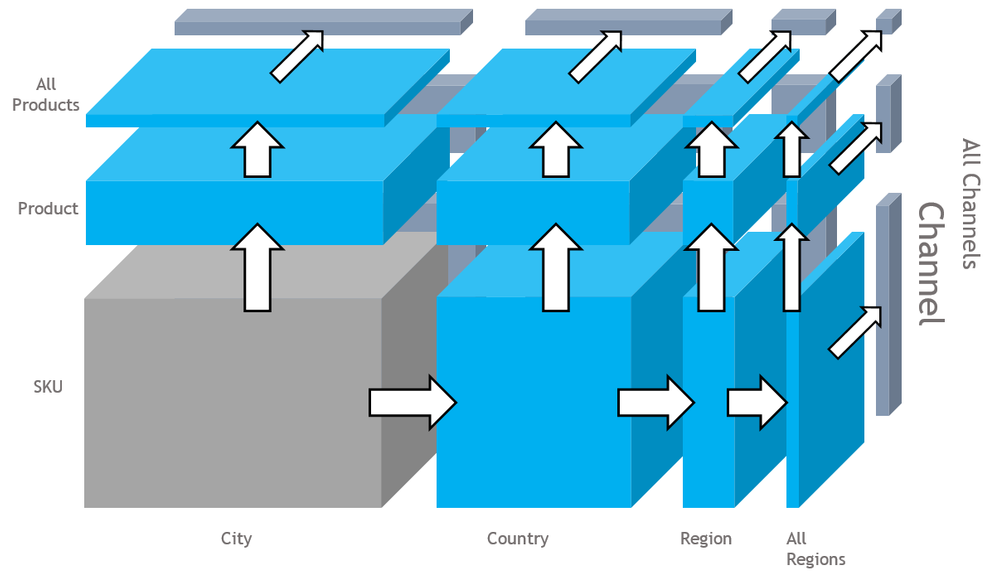With the Summary options set to Sum, when a detailed item is amended (represented in the grey block), all the other aggregations in the hierarchies are also re-calculated. Selecting the None summary option means that no calculations happen when the detail item changes. The varying levels of hierarchies are quite often only there to ease navigation, and the roll-up calculations are not actually needed, so there may be a number of redundant calculations being performed. The native summing of Anaplan is a faster option, but if all the levels are not needed it might be better to turn off the summary calculations and use a SUM formula instead.

For example, from the structure above, let’s assume that we have a detailed calculation for SKU, City, and Channel (SALES06.Final Volume). Let’s also assume we need a summary report by Region and Product, and we have a module (REP01) and a line item (Volume) dimensioned as such.

REP01.Volume = SALES06 Volume Calculation.Final Volume is replaced with REP01.Volume = SALES06.Final Volume[SUM:H01 SKU Details.Product, SUM:H02 City Details.Region]

The second formula replaces the native summing in Anaplan with only the required calculations in the hierarchy.

How do you know if you need the summary calculations? Look for the following:

• Is the calculation or module user-facing?

If it is presented on a dashboard, then it is likely that the summaries will be needed. However, look at the dashboard views used. A summary module is often included on a dashboard with a detail module below; Effectively, the hierarchy sub-totals are shown in the summary module, so the detail module doesn’t need the sum or all the summary calculations.

• Detail to detail

Is the line item referenced by another detailed calculation line item? This is very common, and if the line item is referenced by another detailed calculation the summary option is usually not required. Check the Referenced by column and see if there is anything referencing the line item.

• Calculation and staging modules

If you have used the D.I.S.C.O. module design, you should have calculation/staging modules. These are often not user-facing and have many detailed calculations included in them. They also often contain large cell counts, which will be reduced if the summary options are turned off.

• Can you have different summaries for time and lists?

The default option for Time Summaries is to be the same as the lists. You may only need the totals for hierarchies, or just for the timescales. Again, look at the downstream formulas.

The best practice advice is to turn off the summaries when you create a line item, particularly if the line item is within a Calculation module (from the

## Avoid formula repetition

An optimal model will only perform a specific calculation once. Repeating the same formula expression multiple times will mean that the calculation is performed multiple times. Model builders often repeat formulas related to time and hierarchies. To avoid this, refer to the module design principles (D.I.S.C.O.) and hold all the relevant calculations in a logical place. Then, if you need the calculation, you will know where to find it, rather than add another line item in several modules to perform the same calculation.

If a formula construct always starts with the same condition evaluation, evaluate it once and then refer to the result in the construct. This is especially true where the condition refers to a single dimension but is part of a line item that goes across multiple dimension intersections. A good example of this can be seen in the example below:

START() <= CURRENTPERIODSTART() appears five times and similarly START() > CURRENTPERIODSTART() appears twice.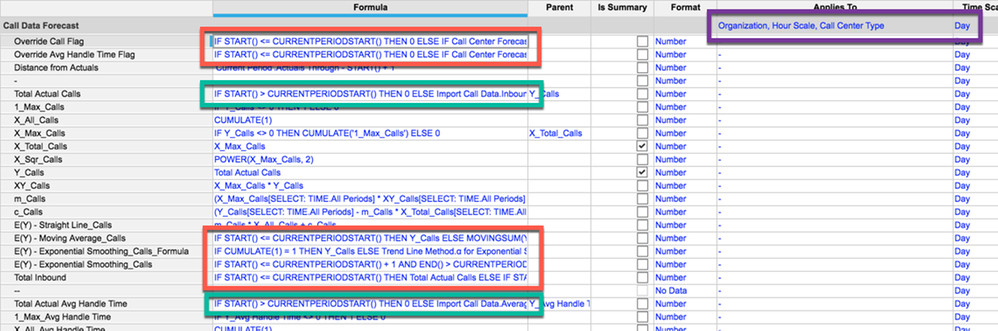To correct this, include these time-related formulas in their own module and then refer to them as needed in your modules.

Remember, calculate once; reference many times!

Taking a closer look at our example, not only is the condition evaluation repeated, but the dimensionality of the line items is also more than required. The calculation only changes by the  day, as per the diagram below: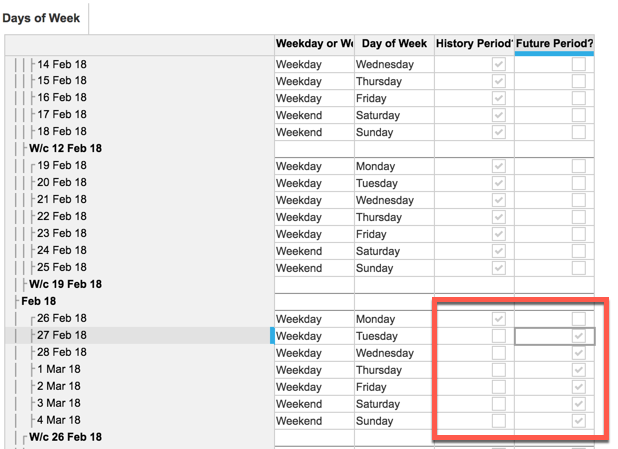But the Applies To here also contains Organization, Hour Scale, and Call Center Type.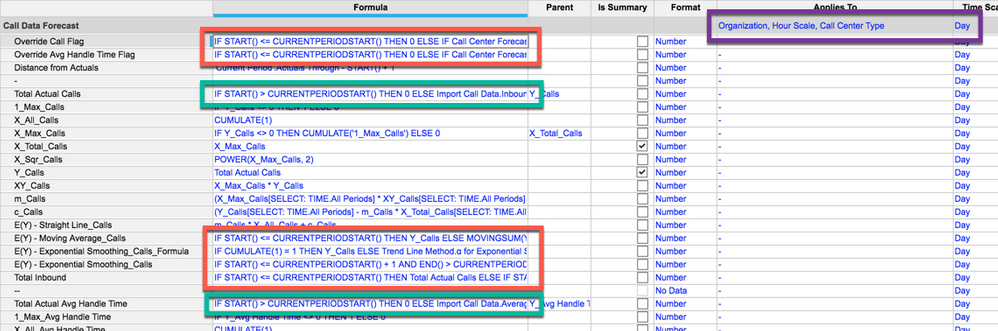Because the formula expression is contained within the line item formula, for each day the following calculations are also being performed: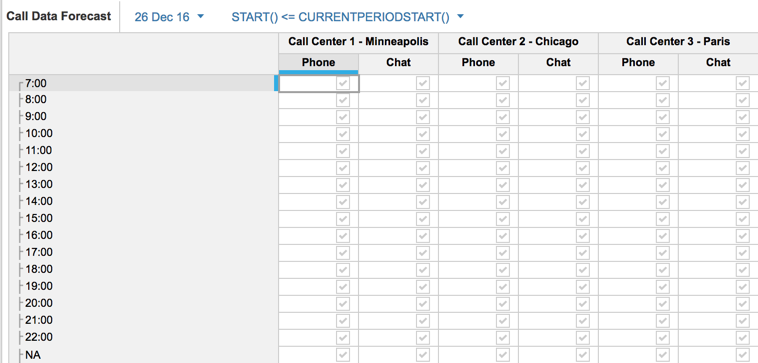And, as above, it is repeated in many other line items.

Sometimes model builders use the same expression multiple times within the same line item. To reduce this overcalculation, reference the expression from a more appropriate module; for example, Days of Week (dimensioned solely by day) which was shown above. The blueprint is shown below, and you can see that the two different formula expressions are now contained in two line items and will only be calculated by day; the other dimensions that are not relevant are not calculated.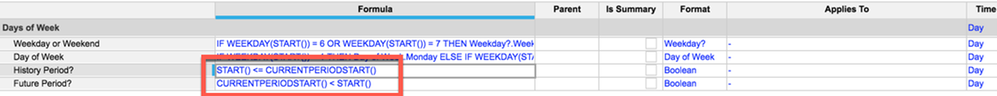Substitute the expression by referencing the line items shown above.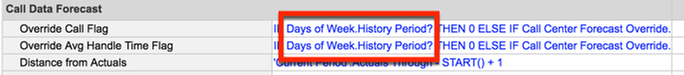In this example, making these changes to the remaining lines in this module reduces the calculation cell count from 1.5 million to 1500.

Check the Applies to for your formulas, and if there are extra dimensions, remove the formula and place it in a different module with the appropriate dimensionality.

Author David Smith.